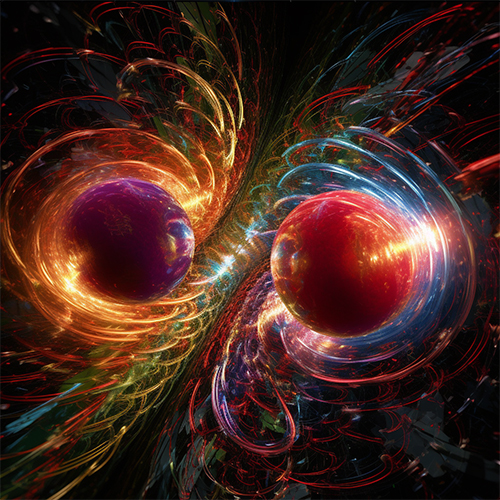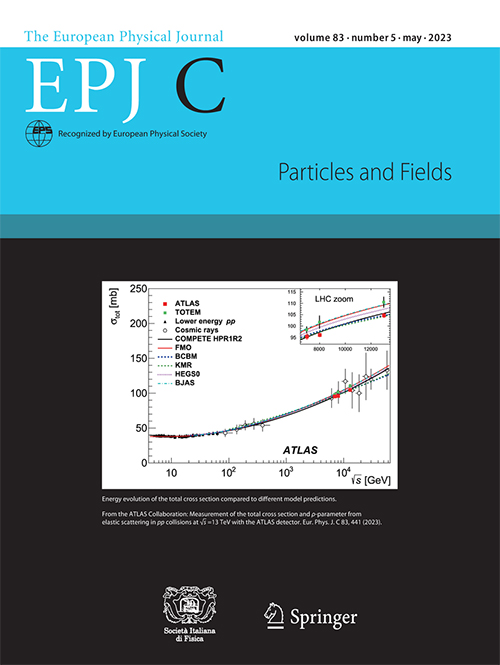﻿ IFJ PAN Press Office

# Quantum proton billiards

10 July 2023Protons accelerated almost to the speed of light can collide similarly to billiard balls. However, since protons are quantum particles, from measuring such collisions we can learn unobvious things about the strong interaction. (Source: IFJ PAN)

The quantum nature of interactions between elementary particles allows drawing non-trivial conclusions even from processes as simple as elastic scattering. The ATLAS experiment at the LHC accelerator reports the measurement of fundamental properties of strong interactions between protons at ultra-high energies.

The physics of billiard ball collisions is taught from early school years. In a good approximation, these collisions are elastic, where both momentum and energy are conserved. The scattering angle depends on how central the collision was (this is often quantified by the impact parameter value – the distance between the centres of the balls in a plane perpendicular to the motion). In the case of a small impact parameter, which corresponds to a highly central collision, the scattering angles are large. As the impact parameter increases, the scattering angle decreases.

In particle physics, we also deal with elastic collisions, when two particles collide, maintaining their identities, and scatter a certain angle to their original direction of motion. Here, we also have a relationship between the collision parameter and the scattering angle. By measuring the scattering angles, we gain information about the spatial structure of the colliding particles and the properties of their interactions.

Physicists from the Institute of Nuclear Physics Polish Academy of Sciences, as part of the ATLAS Collaboration, performed a measurement of elastic scattering in proton–proton collisions at the LHC accelerator at a centre-of-mass energy of 13 TeV. Due to the extremely small scattering angles in such interactions (less than a thousandth of a degree), the measurements required the use of a dedicated measurement system. Its key element was a set of detectors placed over 200 metres from the collision point, but capable of measuring scattered protons at distances of just a few millimetres from the accelerator beam. This was made possible by the technique of so-called Roman pots, which allows placing of detectors inside the accelerator beam pipe and their close approach to the beam during data taking. An important contribution of the Krakow group was the work on the trigger and data acquisition system, without which no data can be recorded.

The second important component of the experimental setup was the special configuration of magnetic fields shaping the LHC accelerator beam. In typical measurements, the goal is to maximise beam focusing in order to increase the frequency of interesting interactions. However, tightly focused beams have a large angular divergence, making the measurement of elastic scattering practically impossible. The special magnet configuration minimises this divergence and ensures precise measurements.

The direct result of the measurement, published in European Physical Journal C, is the distribution of the scattering angle, or more precisely – the distribution of the variable t, which is proportional to the square of that angle. Conclusions regarding the fundamental properties of nuclear strong interactions between protons at very high energies, were drawn from the shape of this distribution. The procedure of extracting this information is based on quantum properties of elastic scattering – effects which are not observed in the game of billiards.

The first of these properties is the so-called optical theorem, which is a consequence of probability conservation in quantum processes. It relates elastic interactions to inelastic ones (i.e. ones where additional particles are produced). Since the protons in the studied collisions have very high energy, inelastic processes occur frequently. The optical theorem allowed determining the value of a parameter called the total cross-section from measurements of only elastic interactions.

The cross-section is a quantity used in particle physics to describe the likelihood of a particular reaction. The total cross-section describes the chance of any type of proton–proton collision and is related to the proton size. The result published by the ATLAS Collaboration is the most precise measurement of this parameter at 13 TeV energy. The high precision was possible, among other factors, by the precise determination of the detector position, for which the IFJ PAN group was responsible. The obtained result confirms an important property of strong interactions – the increase of the total cross-section with increasing collision energy. This increase can be thought of as the proton size increasing with energy.

Having an accurate knowledge of the total cross-section is of interest not only for studying strong interactions themselves but also in other areas of particle physics. Strong interactions are relevant, for example, in the search for new physics in experiments at the LHC, where they act as background, as well as in cosmic ray research, where they are responsible for the development of cosmic air showers. Precise modelling of these processes is possible thanks to precise measurements of quantities such as the total cross-section.

In proton–proton collisions, elastic scattering can occur via two mechanisms: strong nuclear interaction and Coulomb interaction, i.e. the repulsion between electric charges. The second consequence of the quantum nature of the studied process is the interference between these mechanisms. The interference depends on their scattering amplitudes. The scattering amplitude is a measure of probability used in quantum physics. Unlike ordinary probability, its values are not real numbers but complex numbers. Therefore, it is described by either its magnitude and phase or by its real and imaginary parts. Since Coulomb interactions are well understood and their scattering amplitude can be calculated, by measuring the interference, we gain insights into both the real and imaginary parts of the nuclear amplitude.

The experimentally measured value of the ratio of the real to the imaginary parts of the nuclear amplitude turns out to be significantly lower than predictions of pre-LHC theoretical models. These models follow from certain assumptions about the properties of the strong interactions. The observed discrepancy challenges these assumptions.

The first assumption is that at very high energies properties of proton–antiproton collisions are the same as those of proton–proton and antiproton–antiproton collisions. This is because, although protons are made up of quarks and gluons, collisions at high energies only occur predominantly between gluons. Since the gluon structure of protons and antiprotons is the same, the natural assumption is that the interactions in different systems are identical. Allowing a difference, which is possible because of the quantum nature of interactions, makes the theoretical models describe the experimental data.

The second assumption of the theoretical models concerns the growth of the total cross section with energy. It was assumed that its character for energies above those currently measured at the LHC accelerator is the same as observed so far. The observed discrepancy can be explained also by a slowing down of this growth at energies above the LHC energy.

Both considered hypotheses concern the basic properties of the strong interaction at high energies. Regardless of which one is true, the reported measurements shed light on our understanding of fundamental interactions of particles.

At present, the detectors used in the described studies are being prepared for further measurements of elastic scattering at even higher energies. The Institute of Nuclear Physics Polish Academy of Sciences is also conducting research on other processes in which both strong and electromagnetic interactions play significant roles. The technique of Roman pots plays a crucial role in these studies (NCN grant SONATA BIS 2021/42/E/ST2/00350).

[PDF]

#### Contact:

dr Rafał Staszewski
Institute of Nuclear Physics, Polish Academy of Sciences
tel.: +48 12 662 8361
email: rafal.staszewski@ifj.edu.pl

#### Scientific papers:

„Measurement of the total cross-section and ρ-parameter from elastic scattering in pp collisions at √s = 13 TeV with the ATLAS detector”
ATLAS Collaboration;
European Physical Journal C 83 (2023) 441;
DOI: https://doi.org/10.1140/epjc/s10052-023-11436-8

#### Images:Protons accelerated almost to the speed of light can collide similarly to billiard balls. However, since protons are quantum particles, from measuring such collisions we can learn unobvious things about the strong interaction. (Source: IFJ PAN)Cover page of European Physical Journal C journal, Volume 83, Issue 5, May 2023, showing one of the main results of the published analysis – energy evolution of the total proton–proton cross section. (Source: EPJ C/Springer)

﻿